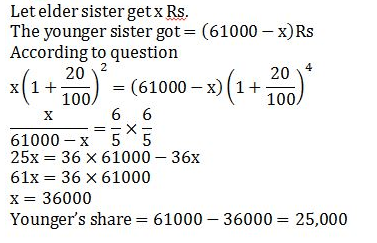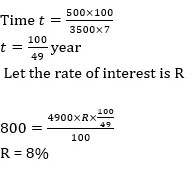# Quant Quiz for IBPS PO & IBPS CLERK PRE

Quant Quiz to improve your Quantitative Aptitude for SBI Po & SBI clerk exam IBPS PO Reasoning , IBPS Clerk Reasoning , IBPS RRB Reasoning, LIC AAO ,  and other competitive exams.

Q1. Madhu invested a certain amount at the rate of 8 p.c.p.a. for 5 years and obtained a simple interest of Rs 3800. Had she invested the same amount at the same rate of interest for 2 years, how much amount would she have obtained as compound interest at the end of 2 years?

A) 1580.80

B) 1520

C) 1550.50

D) 1550

E) None of these

Q1. Ans(a)Q2. The RBI lends a certain amount to the SBI on simple interest for two years at 20%. The SBI gives this entire amount to Bharti Telecom on compound interest for two years at the same rate annually. Find the percentage earning of the SBI at the end of two years on the entire amount.

(a) 4%

(b) 3(1/7)%

(c) 3(2/7)%

(d) 3(6/7)%

(e) None of these

Q2. Ans(a)Q3. A mother divided an amount of Rs. 61,000 between her two daughters aged 18 years and 16 years respectively and deposited their shares in a bond. If the interest rate is 20% compounded annually and if each received the same amount as the other when they attained the age of 20 years, their shares are

(a) Rs. 35,600 and Rs. 25,400

(b) Rs. 30500 each

(c) Rs. 24,000 and Rs. 37000

(d) Rs. 36000  and Rs 25,000

(e) None of these

Q3. AnsQ4. A sum of money lent at compound interest for 2 years at 20% per annum would fetch Rs 723 more, if the interest was payable half-yearly than if it was payable annually. The sum is?

(a) Rs. 38,000

(b) Rs. 30,000

(c) Rs. 20,000

(d) Rs. 25,000

(e) None of these

Q4.  Ans(b)Q5. Manish borrowed some money at the rate of 7 per cent per annul for the first three years, 9 per cent per annul for the next six years and 10 per cent per annul for the period beyond nine years. If the total interest paid by him at the end of fifteen years is Rs 4050, how much money did he borrow( if simple interest be reckoned)?

(a) Rs 2800

(b) Rs 3600

(c) Rs 3000

(d) Rs 3500

(e) None of these

Q5. Ans(c)Q6.  Rakesh borrows Rs. 3500 from a bank at SI. After three years he paid Rs. 1500 to the bank and at the end of 5 years from the date of borrowing he paid Rs. 2725 to the bank to settle the account. Find the rate of interest.

A) 10%

B) 5%

C) 2.5%

D) 3.5%

E) None of these

Q6. Ans(B)Q7. Ashok borrowed some money at simple interest at the rate of 6 per cent per annul for the first two years, at the rate of 9 per cent per annul for the next three years and at the rate of 14% per cent per annul for the period beyond five years. If he pays a total interest of Rs. 11400 at the end of 9 years how much money did he borrow ?

(a) Rs. 16,000

(b) Rs. 14,000

(c) Rs. 18,000

(d) Rs. 12,000

(e) None of these

Q7. Ans(d)Q8. On Rs 3500 invested at a simple interest rate 7 per cent per annum, Rs 500 is obtained as interest in certain years, In order to earn Rs 800 as interest on Rs 4900 in the same number of years, what should be the rate of simple interest?

(a) 9%

(b) 10%

(c) 12%

(d) 8%

(e) None of these

Q8. Ans(d)Q9. A person invested a certain amount at simple interest at the rate of 6 per cent per annul earning Rs. 900 as an interest at the end of three years. Had the interest been compounded every year, how much more interest would he have earned on the same amount with the same interest rate after three years ?

(a) Rs. 38.13

(b) Rs. 25.33

(c) Rs. 55.08

(d) Rs. 35.30

(e) None of these

Q9. Ans(c)Q10. If Rs.1000 be invested at interest rate of 5% and the interest be added to the principle every 10 years, then In how many years  it will amount to Rs. 2000 ?Q10. Ans(a)Best E-books for LIC AAO 2019 : Get PDF here

ambitiousbaba.com need your support to Grow

I challenge you will get Best Content in Our PDFs with Detail solutions and Latest Pattern

Memory Based Puzzle E-book | 2016-19 Exams Covered

Get PDF here

Caselet Data Interpretation 200 Questions

Get PDF here
Puzzle & Seating Arrangement E-Book for BANK PO MAINS (Vol-1)

Get PDF here

### ARITHMETIC DATA INTERPRETATION 2019 E-book

Get PDF here
The Banking Awareness 500 MCQs E-book| Bilingual (Hindi + English)

Get PDF here

High Level DATA INTERPRETATION Practice E-BOOK

Get PDF here

### How to Access on App:-

1. Go to Playstore search Ambitious Baba or Click here to Install App